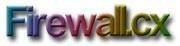# Tips on working with VLSM

## Variable Length Subnet Masks (VLSM) can require some serious number crunching but there are many ways to help make the process simpler.

Variable Length Subnet Masks (VLSM) can require some serious number crunching to figure out when they start getting...

complicated and there are many ways to help make the process simpler.

In my experience of teaching the CCNA course, I have found that every person essentially will find their own way of figuring the fastest method to calculate them as long as they work on it hard enough.Have networking questions? Then visit Firewall.cx, one of the few websites recommended by the Cisco Academy program.

What I can do, hoping to help you, is show you the method I use, so you can then take it and see how well it suites you!

Before we dive into the deep waters, let me show you how I calculate network ranges from given subnet masks:

Assume network 192.168.0.0 and subnet mask 255.255.255.240

I first calculate each subnet's range: 256-240=16 IP's per subnet

This means we have the following subnets:

```0
16
32
48
64
..
...
....
```

As we said, for each subnet, we have 16 IP's this includes network and broadcast addresses. Looking at the above table, I already have the network address for each subnet, so let's reveal the broadcast address:

```0  -  15
16 -  31
32 -  47
48 -  63
64
...
....
```

The logic behind this is quite simple: take the next network and subtracted one from it to get the broadcast address

Now that we have the network and broadcast address, it's easy to calculate the range of VALID IP addresses:

```0   (1 to 14)  15
16  (17 to 30) 31
32  (33 to 46) 47
48  (49 to 62) 63
```

You can clearly see that everything between the network and broadcast address of each subnet is a VALID IP address.

This method is perhaps the best and fastest way to calculate networks and IP ranges from different subnet masks.

One step up is VLSM, where we are given any possible subnet mask and we need to calculate the subnets and IP ranges.

For this, I have created a nice table which is available on my site, under the 'supernetting/CIDR' topic (www.firewall.cx/supernetting-chart.php). The chart shows all possible subnet mask combinations and the corresponding number of networks and hosts calculated for each one.

A closer look at the table will reveal a nice pattern that is repeated:

```Subnet Bits       Subnet mask
/16               255.255.0.0
/17               255.255.128.0
/18               255.255.192.0
/19               255.255.224.0
/20               255.255.240.0
/21               255.255.248.0
/22               255.255.252.0
/23               255.255.254.0
/24               255.255.255.0
/25               255.255.255.128
/26               255.255.255.192
/27               255.255.255.224
```

Notice the subnet mask for /17 and /25, /18 and /26. Both /17 and /25 have the 128 decimal number in their subnet mask, while /18 and /26 have decimal 192. The only part that changes in the pattern is the location of the last 'non-zero' decimal number.

Considering that /8, /16 and /24 have only 255 and 0 decimals, so whenever I see a number between these ranges, I use the closest of the three as a reference point.

For example, /19 is a standard 16 bit subnet mask, with an additional 3 bits. If you memorize the following, then it becomes an easy task translating VLSM numbers to real decimal subnet masks:

```For
1 = 128
2 = 192
3 = 224
4 = 240
5 = 248
6 = 252
7 = 254
```

So, /21 is (2x8)+ 5, that is 255.255.248.0 !

To figure out the subnet masks here, simply do the following calculations:
256-248=8 That's 8 IP's per subnet. We need to reserve 2 IP's (network and broadcast addresses), leaving us with 6 valid IP's per subnet.

From all the above calculations, the hardest part is memorizing the last table, which is only 7 entries. From there on, it's a matter of using the quick method I just showed, to calculate your subnets and their ranges!

Close